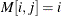# SAS/IML Statistical Functions and CALL Subroutines

The following functions and CALL subroutines compute a variety of statistics or perform mathematical operations that are useful for computing statistics:

Statistical Functions

Function Purpose
BRANKS computes bivariate ranks
CORR computes correlation statistics
COUNTMISS counts the number of missing values
COUNTN counts the number of nonmissing values
COUNTUNIQUE returns the number of unique values
COV computes a sample variance-covariance matrix
CUSUM computes cumulative sums
CUPROD computes cumulative products
DESIGN creates a design matrix
DESIGNF creates a full-rank design matrix
GEOMEAN computes geometric means
HARMEAN computes harmonic means
MAD finds the univariate (scaled) median absolute deviation
MEAN computes sample means
OPSCAL rescales qualitative data to be a least squares fit to qualitative data
RANK ranks elements of a matrix
RANKTIE ranks matrix elements by using tie-averaging
SWEEP sweeps a matrix
VAR computes a sample variance

Statistical CALL Subroutines

CALL Subroutine Purpose
IPF performs an iterative proportional fit of a contingency table
LAV performs linear least absolute value regression by solving thenorm minimization problem
LMS performs robust least median of squares (LMS) regression
LTS performs robust least trimmed squares (LTS) regression
MARG evaluates marginal totals in a multiway contingency table
MAXQFORM computes the subsets of a matrix system that maximize the quadratic form
MCD finds the minimum covariance determinant estimator
MVE finds the minimum volume ellipsoid estimator
QNTL computes sample quantiles (percentiles)
SEQ, SEQSCALE, and EQSHIFT performs discrete sequential tests

Reduction Functions

Function Purpose
MAX finds the maximum value of a matrix
MIN finds the smallest element of a matrix
PROD multiplies all elements
SSQ computes the sum of squares of all elements
SUM sums all elements

Random Number Generation Functions

Function Purpose
NORMAL generates a pseudorandom normal deviate
UNIFORM generates pseudorandom uniform deviates

Random Number Generation CALL Subroutines

CALL Subroutine Purpose
RANDGEN generates random numbers from specified distributions
RANDSEED initializes seed for subsequent RANDGEN calls

You can also call functions in Base SAS software such as those documented in the following sections: